MagnetShop.com## 1.0 Introduction

Magnets are an important part of our daily lives, serving as essential components in everything from electric motors, loudspeakers, computers, compact disc players, microwave ovens and the family car, to instrumentation, production equipment, and research. Their contribution is often overlooked because they are built into devices and are usually out of sight.

Magnets function as transducers, transforming energy from one form to another, without any permanent loss of their own energy. General categories of permanent magnet functions are:

• Mechanical to mechanical - such as attraction & repulsion.
• Mechanical to electrical - such as generators & microphones.
• Electrical to mechanical - such as motors, loudspeakers, charged particle deflection.
• Mechanical to heat - such as eddy current & hysteresis torque devices.
• Special effects - such as magneto resistance, Hall effect devices, & magnetic resonance.

The following sections will provide a brief insight into the design and application of permanent magnets. The Design Engineering team at Magnet Sales & Manufacturing will be happy to assist you further in your applications.

## 2.0 Modern Magnet Materials

There are four classes of modern commercialized magnets, each based on their material composition. Within each class is a family of grades with their own magnetic properties. These general classes are:

NdFeB and SmCo are collectively known as Rare-Earth magnets because they are both composed of materials from the Rare Earth group of elements.

• Neodymium Iron Boron magnets (general composition Nd2Fe14B, often abbreviated to NdFeB) is the most recent commercial addition to the family of modern magnet materials. At room temperatures, NdFeB magnets exhibit the highest properties of all magnet materials.
• Samarium Cobalt magnets are manufactured in two compositions: Sm1Co5 and Sm2Co17 - often referred to as the SmCo 1:5 or SmCo 2:17 types. 2:17 types, with higher Hci values, offer greater inherent stability than the 1:5 types.
• Ceramic (Ferrite) magnets, also known as Ferrite, magnets (general composition BaFe2O3 or SrFe2O3) have been commercialized since the 1950s and continue to be extensively used today due to their low cost. A special form of Ceramic magnet is "Flexible" material, made by bonding Ceramic powder in a flexible binder.
• Alnico magnets (general composition Al-Ni-Co) were commercialized in the 1930s and are still extensively used today.

These magnet materials span a range of properties that accommodate a wide variety of application requirements. The following is intended to give a broad but practical overview of factors that must be considered in selecting the proper material, grade, shape, and size of magnet for a specific application. The chart below shows typical values of the key characteristics for selected grades of various materials for comparison. These values will be discussed in more detail in the below sections.### Table 2.1 Magnet Material Comparisons

Material

Br

Hc

Hci

BHmax

Tmax (ºC)*

NdFeB

39H

12,800

12,300

21,000

40

150

SmCo

26

10,500

9,200

10,000

26

300

NdFeB

B10N

6,800

5,780

10,300

10

150

Alnico

5

12,500

640

640

5.5

540

Ferrite

8

3,900

3,200

3,250

3.5

300

Flexible

1

1,600

1,370

1,380

0.6

1000

*Tmax (maximum practical operating temperature) is for reference only. The maximum practical operating temperature of any magnet is dependent on the circuit the magnet is operating in.

## 3.0 Units Of Measure

Three systems of units of measure are common: the cgs (centimeter, gram, second), SI (meter, kilogram, second), and English (inch, pound, second) systems. This catalog uses the cgs system for magnetic units, unless otherwise specified.### Table 3.1 Units of Measure System

#### English System

Flux

%

maxwell

weber

maxwell

Flux Density

B

gauss

tesla

lines/in2

Magnetomotive
Force

F

gilbert

ampere
turn

ampere
turn

Magnetizing
Force

H

oersted

ampere
turns/m

ampere
turns/in

Length

L

cm

m

in

Permeability of a vacuum

µv

1

0.4π  x 10-6

3.192### Table 3.2 Conversion Factors

Multiply

By

To Obtain

Inches

2.54

Centimeters

Lines/in2

0.155

Gauss

Lines/in2

1.55 x 10-5

Tesla

Gauss

6.45

Lines/in2

Gauss

0-4

Tesla

Gilberts

0.79577

Ampere turns

Oersteds

79.577

Ampere turns/m

Ampere turns

0.4"

Gilberts

Ampere turns/in

0.495

Oersteds

Ampere turns/in

39.37

Ampere turns/m

Mega Gauss Oersteds

7.91667

Kilo Joules/m3

## 4.0 Design Considerations

Basic problems of permanent magnet design revolve around estimating the distribution of magnetic flux in a magnetic circuit, which may include permanent magnets, air gaps, high permeability conduction elements, and electrical currents. Exact solutions of magnetic fields require complex analysis of many factors, although approximate solutions are possible based on certain simplifying assumptions. Obtaining an optimum magnet design often involves experience and trade offs.

### 4.1 Finite Element AnalysisFinite Element Analysis (FEA) modeling programs are used to analyze magnetic problems in order to arrive at more exact solutions, which can then be tested and fine tuned against a prototype of the magnet structure. Using FEA models flux densities, torques, and forces may be calculated. Results can be output in various forms, including plots of vector magnetic potentials, flux density maps, and flux path plots. Our design and engineering team at our sister company, Integrated Magnetics has extensive experience in many types of magnetic designs and is able to assist in the design and execution of FEA models.

### 4.2 The B-H Curve

The basis of magnet design is the B-H curve, or hysteresis loop, which characterizes each magnet material. This curve describes the cycling of a magnet in a closed circuit as it is brought to saturation, demagnetized, saturated in the opposite direction, and then demagnetized again under the influence of an external magnetic field..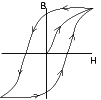The second quadrant of the B-H curve, commonly referred to as the "Demagnetization Curve", describes the conditions under which permanent magnets are used in practice. A permanent magnet will have a unique, static operating point if air-gap dimensions are fixed and if any adjacent fields are held constant. Otherwise, the operating point will move about the demagnetization curve, the manner of which must be accounted for in the design of the device.

The three most important characteristics of the B-H curve are the points at which it intersects the B and H axes (at Br - the residual induction - and Hc - the coercive force - respectively), and the point at which the product of B and H are at a maximum (BHmax - the maximum energy product). Br represents the maximum flux the magnet is able to produce under closed closed circuit conditions. In actual useful operation, permanent magnets can only approach this point. Hc represents the point at which the product of B and H, and the energy density of the magnetic field into the air gap surrounding the magnet, is at a maximum. The higher this product, the smaller the volume of the magnet need be. Designs should also account for the variation of the B-H curve with temperature. This effect is more closely examined in the section entitled," Permanent Magnet Stability.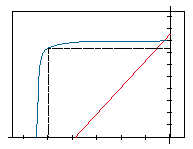When plotting a B-H curve, the value of B is obtained by measuring the total flux in the magnet (φ) and then dividing this by the magnet pole area (A) to obtain the flux density (B=φ/A). The total flux is composed of the flux produced in the magnet by the magnetizing field (H), and the intrinsic ability of the magnet material to produce more flux due to the orientation of the domains. The flux density of the magnet is therefore composed of two components, one equal to the applied H, and the other created by the intrinsic ability of ferromagnetic materials to produce flux. The intrinsic flux density is given the symbol B1 where total flux B = H + B1 or B1 = B-H.

In normal operating conditions, no external magnetizing field is present, and the magnet operates in the second quadrant, where H has a negative value. Although strictly negative, H is usually referred to as a positive number, and therefore, in normal practice, B = B + H. It is possible to plot an intrinsic as well as a normal B-H curve. The point at which the intrinsic curve crosses the H axis is the intrinsic coercive force, and is given the symbol Hc1, High Hc1 values are an indicator of inherent stability of the magnet material. The normal curve can be derived from the intrinsic curve and vice versa. In practice, if a magnet is operated in a static manner with no external fields present, the normal curve is sufficient for design purposes. When external fields are present, the normal and intrinsic curves are used to determine the changes in the intrinsic properties of the material.

### 4.3 Magnet Calculations

In absence of any coil excitation, the magnet length and pole area may be determined by the following equations: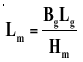Equation 1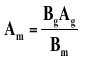Equation 2

Where,

• Bm = the flux density at the operating point,
• Hm = the magnetizing force at the operating point,
• Ag = the air-gap area,
• Lg = the air-gap length,
• Bg = the gap flux density,
• Am = the magnet pole area,
• Lm = the magnet length.

Combining the two equations, the permeance coefficient Pc may be determined as follows: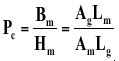Equation 3

Strictly,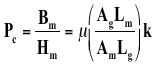Where µ is the permeability of the medium, and k is a factor which takes account of leakage and reluctance that are functions of the geometry and composition of the magnetic circuit.

(The intrinsic permeance coefficient Pci = Bi/H. Since the normal permeance coefficient Pc = B/H, and B = H + Bi, Pc = (H + Bi)/H or Pc = 1 + Bi /H. Even though the value of H in the second quadrant is actually negative, H is conventionally referred to as a positive number. Taking account of this convention, Pc = 1 - Bi/H, or Bi/H = Pci = Pc + 1. In other words, the intrinsic permeance coefficient is equal to the normal permeance coefficient plus 1. This is a useful relationship when working on magnet systems that involve the presence of external fields).

We can compare the various magnet materials for general characteristics using equation 3 above.

Consider that a particular field is required in a given air-gap, so that the parameters Bg, Hg (air-gap magnetizing force), Ag, and Lg are known.

• Alnico 5 has the ability to provide very high levels of flux density Bm, which is often desirable in high performance electromechanical devices. This is accompanied, however, by a low coercivity Hm, and so some considerable magnet length will be required.
• Alnico 8 operates at a higher magnetizing force, Hm, needing a smaller length Lm, but will yield a lower Bm, and would therefore require a larger magnet area Am.
• Rare Earth Magnet Materials offer reasonable to high values of flux density at very high values of magnetizing force. Consequently, very short magnet lengths are needed, and the required volume of this material will be small.
• Ferrite operates at relatively low flux densities, and will therefore need a correspondingly greater pole face area, Am.

The permeance coefficient method using the demagnetization curves allows for initial selection of magnet material, based upon the space available in the device, this determining allowable magnet dimensions.

### 4.3.1 Calculations of Flux Density on a Magnet's Central Line

Use our On-Line Calculators to calculate flux density of rectangular or cylindrical magnets in various configurations.

For Magnet Materials with straight-line normal demagnetization curves such as Rare Earth and Ceramic magnets, it is possible to calculate with reasonable accuracy the flux density at a distance X from the pole surface (where X>0) on the magnet's centerline under a variety of conditions.

#### a. Cylindrical Magnet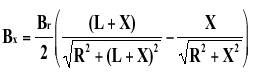Equation 4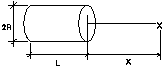Table 4.3.1 shows flux density calculations for a magnet 0.500" in diameter by 0.250" long at a distance of 0.050" from the pole surface, for various materials. Note that you may use any unit of measure for dimensions; since the equation is a ratio of dimensions, the result is the same using any unit system. The resultant flux density is in units of gauss.### Table 4.1 Flux Density vs. Material

Residential Flux Density, Br

Flux at Distance of 0.050" from surface of magnet

Ceramic 1

2,200

629

Ceramic 5

3,950

1,130

SmCo 18

8,600

2,460

SmCo 26

10,500

3,004

NdFeB 35

12,300

3,518

NdFeB 42H

13,300

3,804

#### b. Rectangular Magnets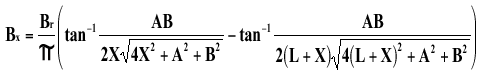Equation 5 (where all angles are in radians)

#### c. Ring Shaped Magnets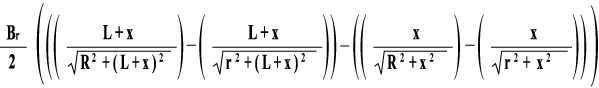Equation 6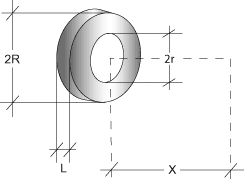#### d. For a magnet on a Steel Back Plate

Substitute 2L for L in case 4, and adopt the same precedure to calculate BP.

#### e. For Identical Magnets Facing Each Other in Attracting Positions

The value of Bx at the gap center is double the value of Bx in case 3. At a point P, Bp is the sum of B(x-p) and B(x-p), where (X+P) and (X-P) substitute for X in case 3.

#### f. For Identical, Yoked Magnets Facing Each Other in Attracting Positions

Substitute 2L for L in case 4, and adopt the same procedure to calculate Bp.

### Table 4.3.2 Force Calculations

The attractive force exerted by a magnet to a ferromagnetic material may be calculated by: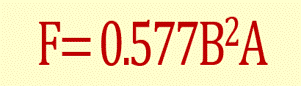Equation 7

Where F is the force in pounds, B is the flux density in Kilogauss, and A is the pole area in square inches. Calculating B is a complicated task if it is to be done in a rigorous manner. However, it is possible to approximate the holding force of certain magnets in contact with a piece of steel using the relationship: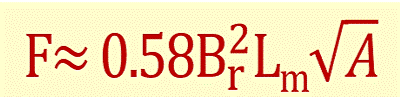Equation 8

Where Br is the residual flux density of the material, A is the pole area in square inches, and Lm ;is the magnetic length. Click here to calculate the Approximate Pull of a rectangular or disc magnet. This formula is only intended to give an order of magnitude for the holding force that is available from a magnet with one pole in direct contact with a flat, machined, steel surface. The formula can only be used with straight-line demagnetization curve materials - i.e. for rare earth and ferrite materials - and where the magnet length, Lm, is kept within the bounds of normal, standard magnet configurations.

## 5.0 Permanent Magnet Stability

The ability of a permanent magnet to support an external magnetic field results from small magnetic domains "locked" in position by crystal anisotropy within the magnet material. Once established by initial magnetization, these positions are held until acted upon by forces exceeding those that lock the domains. The energy required to disturb the magnetic field produced by a magnet varies for each type of material. Permanent magnets can be produced with extremely high coercive forces (Hc) that will maintain domain alignment in the presence of high external magnetic fields.

Stability can be described as the repeated magnetic performance of a material under specific conditions over the life of the magnet. Factors affecting magnet stability include time, temperature, reluctance changes, adverse fields, radiation, shock, stress, and vibration.

### 5.1 Time

The effect of time on modern permanent magnets is minimal. Studies have shown that permanent magnets will see changes immediately after magnetization. These changes, known as "magnetic creep", occur as less stable domains are affected by fluctuations in thermal or magnetic energy, even in a thermally stable environment. This variation is reduced as the number of unstable domains decreases. Rare Earth Magnets are not as likely to experience this effect because of their extremely high coercivities. Long-term time versus flux studies have shown that a newly magnetized magnet will lose a minor percent of its flux as a function of age. Over 100,000 hours, these losses are in the range of essentially zero for Samarium Cobalt magnet materials to less than 3% for Alnico 5 magnet materials at low permeance coefficients.

### 5.2 Temperature

Temperature effects fall into three categories

• Reversible losses.
• Irreversible but recoverable losses.
• Irreversible & unrecoverable losses.

### 5.2.1 Reversible Losses

These are losses that are recovered when the magnet returns to its original temperature. Reversible losses cannot be eliminated by magnet stabilization. Reversible losses are described by the Reversible Temperature Coefficients (Tc), shown in table 5.1. Tc is expressed as % per degree Centigrade. These figures vary for specific grades of each material but are representative of the class of material as a whole. It is because the temperature coefficients of Br and Hc are significantly different that the demagnetization curve develops a "knee" at elevated temperatures.

### Table 5.2.1 Reversible Temperature Coefficients of Br and Hc

 Material Tc of Br Tc of Hc NdFeB -0.12 -0.6 SmCo -0.04 -0.3 Alnico -0.02 0.01 Ceramic -0.2 0.3

### 5.2.2 Irreversible but Recoverable Losses

These losses are defined as partial demagnetization of the magnet from exposure to high or low temperatures. These losses are only recoverable by re-magnetization, and are not recovered when the temperature returns to its original value. These losses occur when the operating point of the magnet falls below the knee of the demagnetization curve. An efficient permanent magnet design should have a magnetic circuit in which the magnet operates at a permeance coefficient above the knee of the demagnetization curve at expected elevated temperatures. This will prevent performance variations at elevated temperatures.

### 5.2.3 Irreversible and Unrecoverable Losses

Metallurgical changes occur in magnets exposed to very high temperatures and are not recoverable by re-magnetization. Table 5.2.3 shows critical temperatures for the various materials where:

• Tcurie is the Curie temperature at which the elementary magnetic moments are randomized and the material is demagnetized.
• Tmax is the maximum practical operating temperature for general classes of major magnetic materials. Different grades of each material exhibit values differing slightly from the values shown here.

### Table 5.2.3 Critical Temperatures for Various Materials

 Material Tcurie *ºC (º F) Tmax *ºC (ºF) Neodymium Iron Boron 310ºC (590ºF) 150ºC (302ºF) Samarium Cobalt 750ºC (1382ºF) 300ºC (572ºF Alnico 860ºC (1580ºF) 540ºC (1004ºF) Ceramic 460ºC (860ºF) 300ºC (572ºF)
*temperatures are shown in degrees Centigrade with the Fahrenheight equivalent in parenthesis.

* Note that the maximum practical operating temperature is dependent on the operating point of the magnet in the circuit. The higher the operating point on the Demagnetization Curve, the higher the temperature at which the magnet may operate.

Flexible magnet materials are not included in this table since the binders that are used to render the magnetic flexible break down before metallurgical changes occur in the magnetic ferrite powder that provides flexible magnets with their magnetic properties.

Partially demagnetizing a magnet by exposure to elevated temperatures in a controlled manner stabilizes the magnet with respect to temperature. The slight reduction in flux density improves a magnet's stability because domains with low commitment to orientation are the first to lose their orientation. A magnet thus stabilized will exhibit constant flux when exposed to equivalent or lesser temperatures. Moreover, a batch of stabilized magnets will exhibit lower variation of flux when compared to each other since the high end of the bell curve which characterizes normal variation will be brought in closer to the rest of the batch.

These changes occur when a magnet is subjected to permeance changes such as changes in air gap dimensions during operation. These changes will change the reluctance of the circuit, and may cause the magnet's operating point to fall below the knee of the curve, causing partial and/or irreversible losses. The extents of these losses depend upon the material properties and the extent of the permeance change. Stabilization may be achieved by pre-exposure of the magnet to the expected reluctance changes.